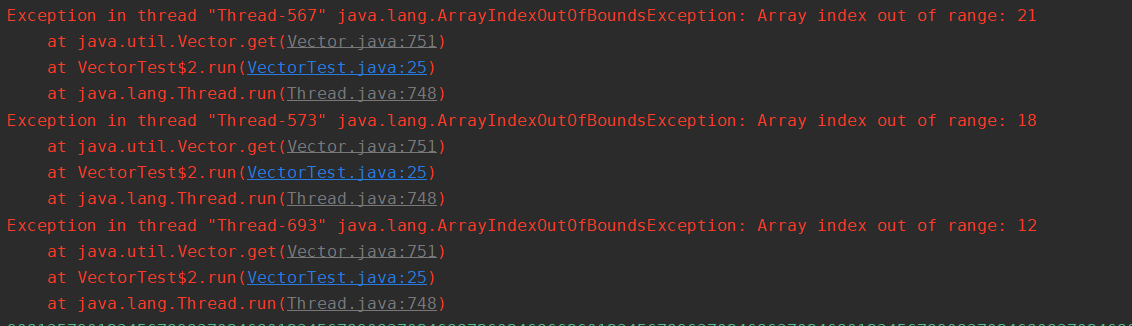## Java中的线程安全的理解

### 绝对线程安全

``````import java.util.Vector;

public class VectorTest {

private static Vector<Integer> vector = new Vector<>();

public static void main(String[] args) {
while (true) {
for (int i = 0; i < 10; i++) {
}
@Override
public void run() {
for (int i = 0; i < vector.size(); i++) {
vector.remove(i);
}
}
});

@Override
public void run() {
for (int i = 0; i < vector.size(); i++) {
System.out.print(vector.get(i));
}
}
});

//                return;
}
}
}

}

``````1、互斥同步

## 锁优化

### 锁消除

``````public String concatString(String s1,String s2,String s3){
StringBuffer sb = new StringBuffer();
sb.append(s1);
sb.append(s2);
sb.append(s3);
return sb.toString();
}
``````

### 锁粗化

``````for(int i=0;i<10;i++){
synchronized(lock){
// do something
}
}
``````

`````` synchronized(lock){
for(int i=0;i<10;i++){
// do something
}
}
``````

### 锁升级的过程

`-XX:-UseBiasedLocking=false`

Mark Word：32比特空间，25位是HashCode，4比特是GC分代年龄，2比特是用来存储锁标志，1比特固定为0

https://juejin.im/post/5ca766dcf265da30d02fb35c